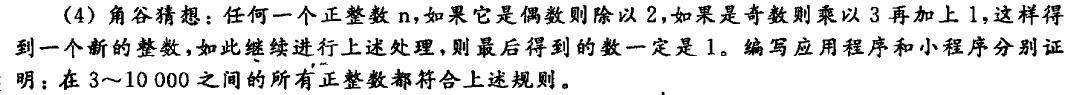# java上机实验

## 角谷猜想### 分析

• 题干中有相关判断的关键字：“如果” ，所以我们需要判断语句
• 然后判断完有俩个分支，一个偶数，一个奇数，因此需要分别编写每种情况的处理方式。
• 题干还有一个关键字：“如此继续”，所以我们还需要循环语句
• 最后题干最后给出了循环判断方式：“得到的数一定是1”，因此作为循环跳出条件。

### 暴力破解

1. 下面是普通模式
public class jiao_gu {
public static void main(String[] arg)
{
int a = 10000; //a 可以是3~10000之间的任意整数，牛逼的可以自己实现输入。
while(a != 1)
{
if(a % 2 == 0)
{
a /= 2;
}else{
a = a * 3 + 1;
}
System.out.println(a);
}
}
}

1. 下面是小程序模式
import java.awt.*;
import java.applet.*;
//虽然Applet在编译器里显示过时了，但我们为了作业，忍了！
public class jiaogu_applet extends Applet
{
Label lab;
TextField input;
int num;

public void init()
{
lab = new Label("Please input a Number(3-10000):");
input = new TextField(5);

}

public boolean action(Event e, Object o)
{
if (e.target == input)
{
num = Integer.parseInt(input.getText());
while(num != 1)
{
if(num % 2 == 0)
{
num /= 2;
}else{
num = num * 3 + 1;
}
}showStatus( "The Final Num:" + num);
}
return true;
}
}

••